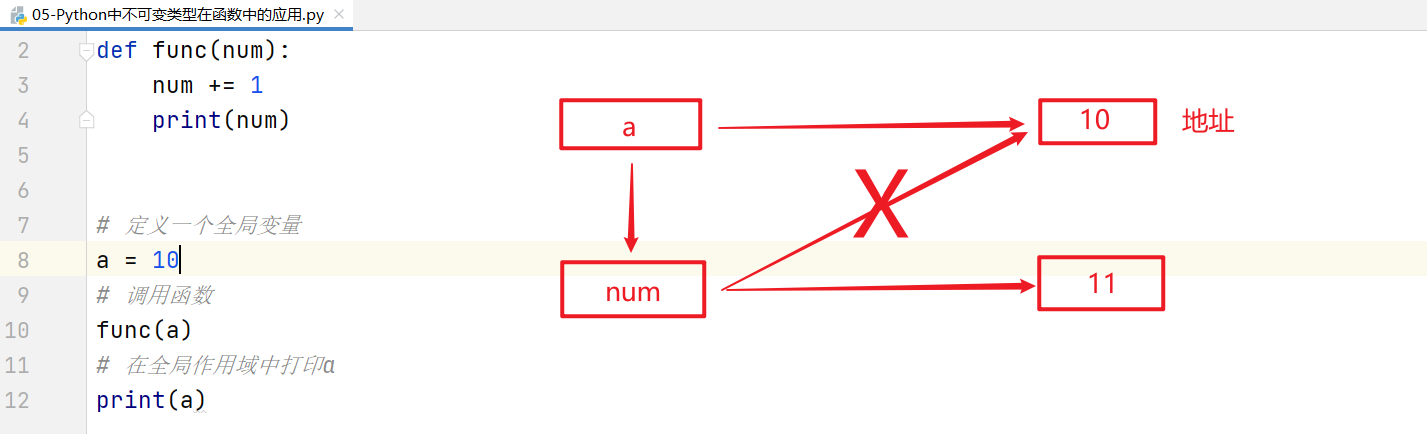WHCSRL 技术网

# python基础学习笔记11：函数进阶

## 1、引用变量

### ☆ 聊聊变量在内存底层的存储形式

``````a = 10
```1```
• 第一步：首先在计算机内存中创建一个数值10（占用一块内存空间）
• 第二步：在栈空间中声明一个变量，如`a`
• 第三步：把数值10的内存地址赋予给变量小a，形成所谓的引用关系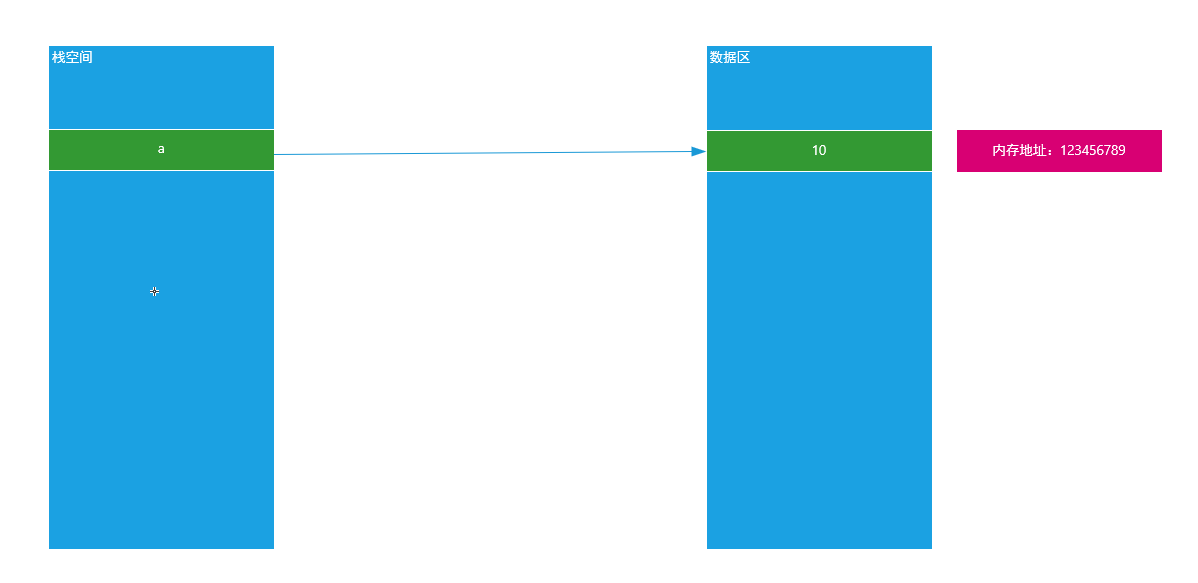### ☆ 如何验证Python中变量的引用关系

``````a = 10
print(id(a))
```12```

### ☆ 把一个变量赋予给另外一个变量的影响

``````a = 10
b = a
print(id(a))
print(id(b))
```1234```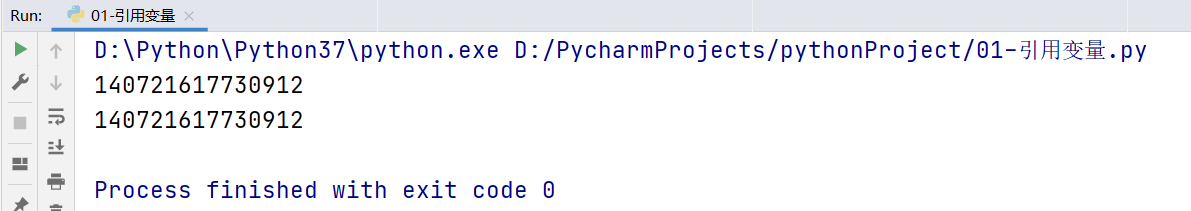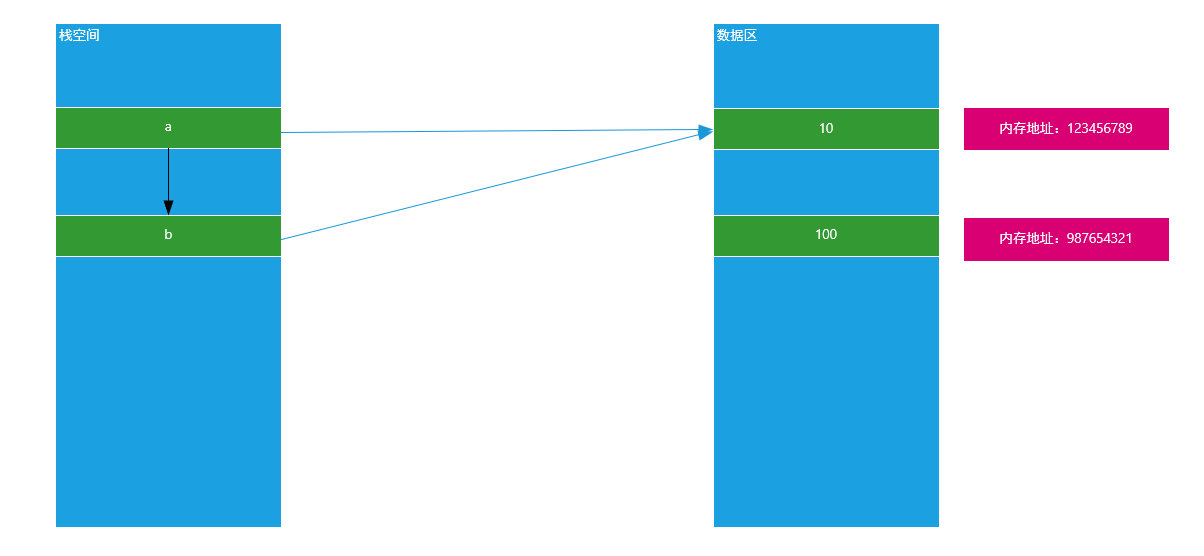``````# a = 10
# print(id(a))

a = 10
b = a

a = 100
print(b)  # 10 或 100

print(id(a))
print(id(b))
```1234567891011```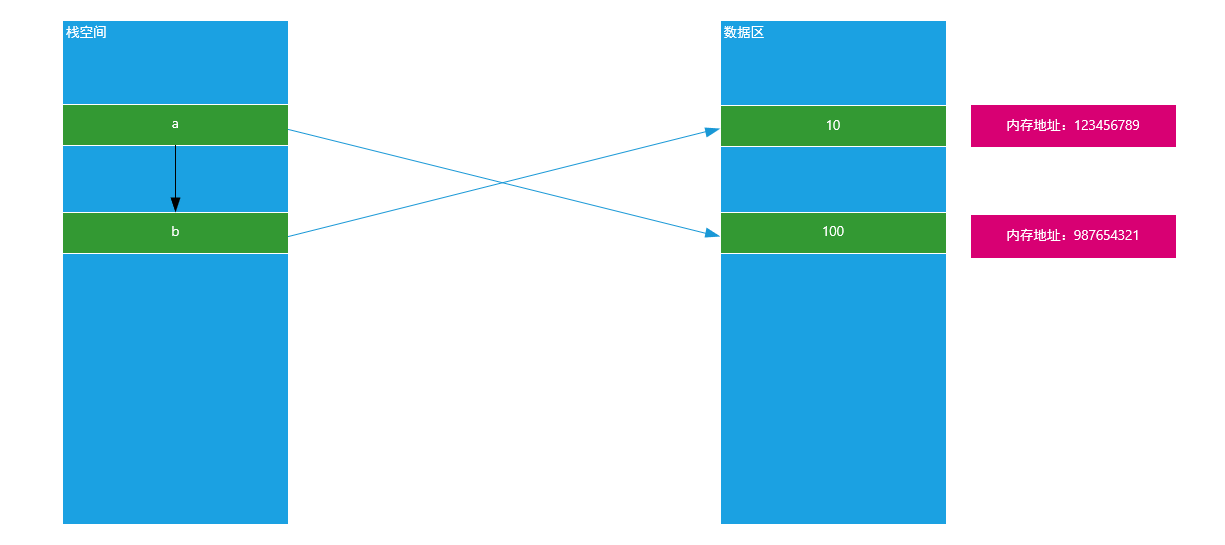## 2、Python中可变和非可变数据类型

☆在Python中一共有几种数据类型？

7种：

• 数值：int整型、float浮点类型
• bool类型：True和False
• 字符串类型：str
• 元组 tuple： (1,2,3）
• 列表 list： [1, 2, 3]
• 字典 dict： {key:value}
• 集合 set： {1, 2}

• 非可变类型

• bool类型：True和False
• 字符串类型：str
• 元组 tuple： (1,2,3）
• 可变类型

• 列表 list： [1, 2, 3]
• 字典 dict： {key:value}
• 集合 set： {1, 2}

☆ 如何判断一个数据类型是可变类型还是非可变类型？

• 可变类型就是在内存中，其内存地址一旦固定，其值是可以发生改变的
``````a = [1, 2, 3]
print(id(a))

# 向内存中追加新数据
a.append(4)
print(id(a))
```123456```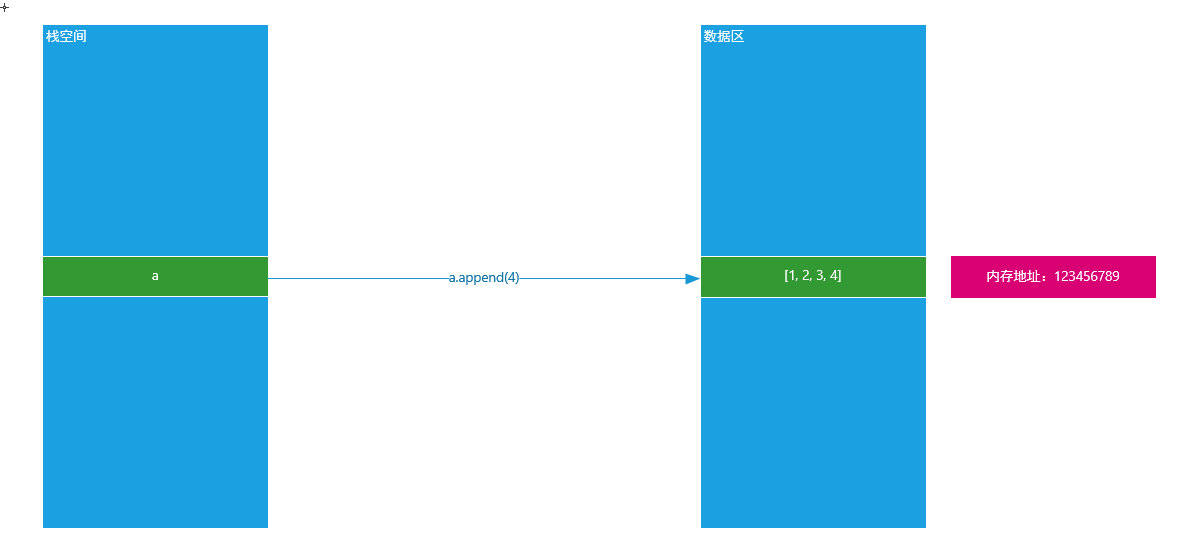• 非可变类型就是在内存中，内存地址一旦固定，其值就没办法发生任何改变了
``````a = 10
print(id(a))

a = 'hello'
print(id(a))
```12345```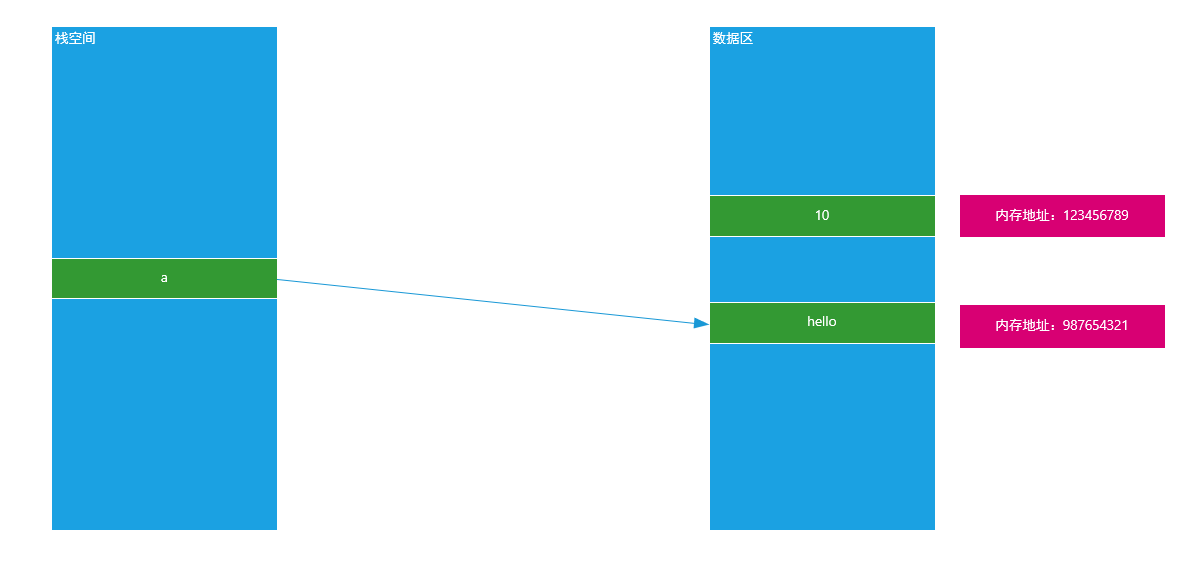## 3、可变类型与非可变类型在函数中的应用

``````# 定义一个函数
def func(names):
print(names)

# 定义一个全局变量
names = ['张三', '李四', '王五']
# 调用函数
func(names)
```12345678```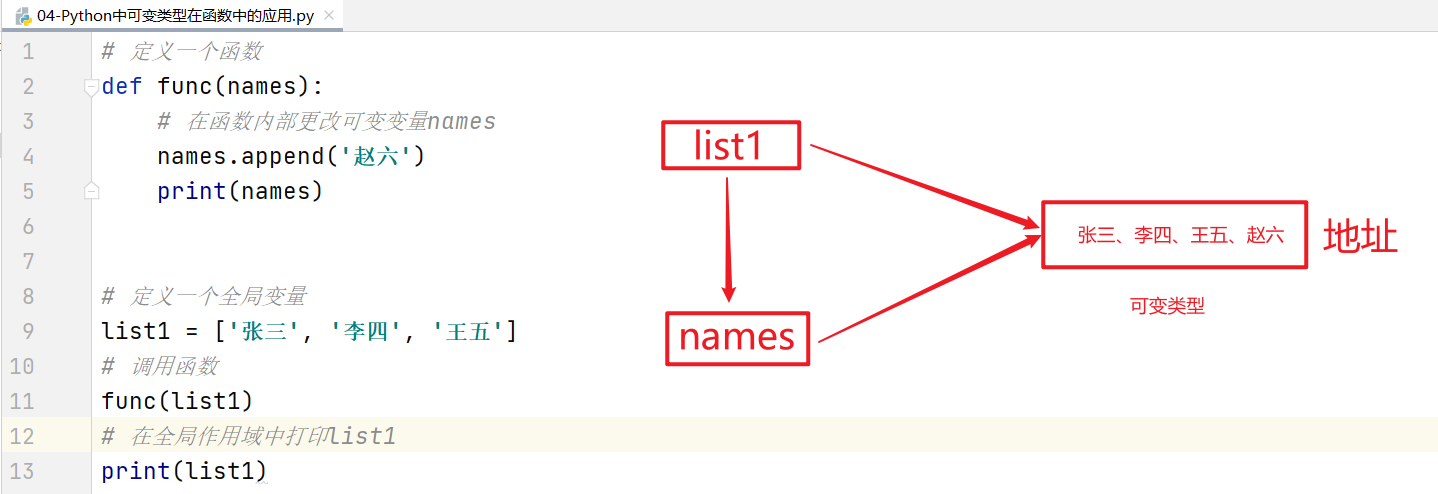☆ 不可变类型

``````# 定义一个函数
def func(num):
num += 1
print(num)

# 定义一个全局变量
a = 10
# 调用函数
func(a)
# 在全局作用域中打印a
print(a)
```1234567891011```